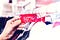# Descriptive Statistics Part 5

In this tutorial, we will discuss about percentiles , quantiles and quantiles in data .Photo by Artem Beliaikin on Unsplash

So , In data how can we find where is 10 percentiles , where is 20 , 30 so on.

In section 1, will discuss about percentiles .

In section 2, will discuss about quantiles or quartiles in depth .

So, understand in depth let take a simple sample of height data -

Height distribution — 18 , 19 , 20, 55 , 48 , 46, 77, 15, 17, 66.

It’s height of sample . now visualize the quantiles and percentiles.

First of all, we…## Puneet Singh

Data Science and Machine Learning Enthusiast.